# Engineering Mechanics CBCGS 2018-2019 BE IT (Information Technology) Semester 1 (FE First Year) Question Paper Solution

Engineering Mechanics [CBCGS]
Date: December 2018

NB:

1.Question No 1 is complusory. Out of the remaining 5 questions, attempt any 3.

2. Numbers in square brackets, at the end of question statement, indicate full marks.

3.Assume suitable data. if not given Clearly mention the same in your answer,

4. Take the magnitude of gravitational acceleration as 9.81m/s2, unless stated otherwise .

5. Figures drawn are not to the scale.

 1
 1.a

Find the resultant of the parallel force system shown in Figure 1 and locate the same with respect to point C.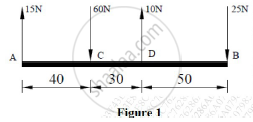Concept: Resultant of parallel forces
Chapter:  System of Coplanar Forces
 1.b

Using Instantaneous Centre of Rotation (ICR) method, find the velocity of point A for the instant shown in Figure 2. Collar B moves along the vertical rod, whereas link AB moves along the plane which is inclined at 250. Ɵ = 450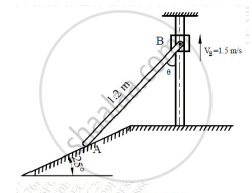Concept: Instantaneous Center of Rotation for the Velocity
Chapter:  Kinematics of Rigid Bodies
 1.c

If the support reaction at A, for the beam shown in Figure 3, is zero, then find force ‘P’ and the support reaction at B.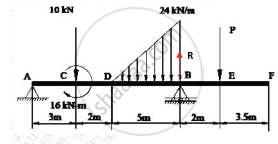Concept: Determination of reactions at supports for various types of loads on beams
Chapter:  Types of Support
 1.d

From the top of a tower, 28 m high, a stone is thrown vertically up with a velocity of 9m/s. After how much time will the stone reach the ground? With what velocity does it strike the ground?

Concept: Velocity Diagrams for Bodies in Plane Motion.
Chapter:  Kinematics of Rigid Bodies
 1.e

For the truss shown in figure 4, find: (i) zero force members, if any (Justify your answer with FBD), (ii) support reactions at C and D.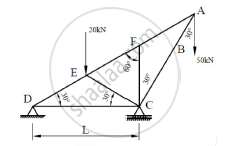Chapter:  Types of Support
 2
 2.a

For the composite lamina shown in the figure, determine the coordinates of its centroid.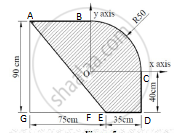Concept: Centroid for Plane Laminas
Chapter:  Center of Gravity and Centroid for Plane Laminas
 2.b
Replace the force system shown in Figure 6 with a single force and couple system acting at point B.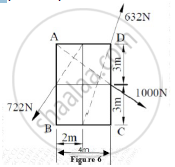Concept: Resultant of Concurrent Force System
Chapter: [6.01] Resultant of Noncoplanar force systems
 2.c

The link CD of the mechanism shown in Figure 7 is rotating in counterclockwise direction at an angular velocity of 5 rad/s. For the given instance, determine the angular velocity of link AB.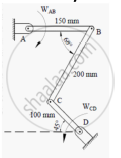Concept: Instantaneous Center of Rotation for the Velocity
Chapter:  Kinematics of Rigid Bodies
 3
 3.a

Cylinder A (diameter 1m, weight 20 kN) and cylinder B (diameter 1.5m, weight 40 kN) are arranged as shown in Figure 8. Find the reactions at all contact points. All contacts are smooth.Concept: Equilibrium of parallel force system
Chapter: [6.02] Equilibrium of Noncoplanar force systems
 3.b

Using Principle of Virtual Work, determine the force P which will keep the weightless bar AB in equilibrium. Take length AB as 2m and length AC as 8m. The bar makes an angle of 30° with horizontal. All the surfaces in contact are smooth. Refer Figure 9.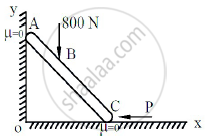Concept: Applications on Equilibrium Mechanisms
Chapter:  Principle of Virtual Work
 3.c

Velocity-time diagram for a particle travelling along a straight line is shown in Figure 10. Draw acceleration-time and displacement-time diagram for the particle. Also find important values of acceleration and displacement.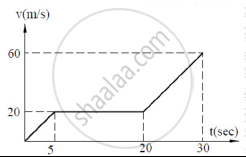Concept: Velocity and Acceleration in Terms of Rectangular Co-ordinate System
Chapter:  Kinematics of Particle
 4
 4.a

Find the force ‘F’ to have motion of block A impeding up the plane. Take coefficient of friction for all the surfaces in contact as 0.2. Consider the wedge B as weightless. Refer Figure 11.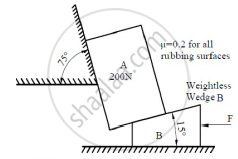Concept: Application to Problems Involving Wedges
Chapter:  Friction
 4.b

Three forces F1, F2 and F3 act at the origin of Cartesian coordinate axes system. The force F1 (= 70N) acts along OA whereas F2 (= 80N) acts along OB and F3 (= 100N) acts along OC. The coordinates of the points A, B and C are (2,1,3), (-1,2,0) and (4,-1,5) respectively. Find the resultant of this force system.

Concept: Resultant of Concurrent Force System
Chapter: [6.01] Resultant of Noncoplanar force systems
 4.c

A 75kg person stands on a weighing scale in an elevator. 3 seconds after the motion starts from rest, the tension in the hoisting cable was found to be 8300N. Find the reading of the scale, in kg during this interval. Also find the velocity of the elevator at the end of this interval. The total mass of the elevator, including mass of the person and the weighing scale, is 750kg. If the elevator is now moving in the opposite direction, with same magnitude of acceleration, what will be the new reading of the scale?

Concept: Velocity and Acceleration in Terms of Rectangular Co-ordinate System
Chapter:  Kinematics of Particle
 5
 5.a

The cylinder B, diameter 400mm and weight 5kN, is held in position as shown in Figure 12 with the help of cable AB. Find the tension in the cable and the reaction developed at contact C.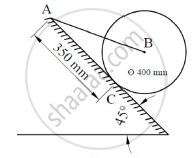Concept: Determination of reactions at supports for various types of loads on beams
Chapter:  Types of Support
 5.b

Find the weight WB so as to have its impending motion down the plane. Take weight of block A as 2kN. The pin connected rod AB is initially is in horizontal position. Refer Figure 13. Coefficient of friction = 0.25 for all surfaces.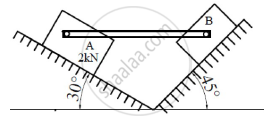Concept: Cone of Friction
Chapter:  Friction
 5.c

Two springs, each having stiffness of 0.6N/cm and length 20 cm are connected to a ball B of weight 50N. The initial tension developed in each spring is 1.6N. The arrangement is initially horizontal, as shown in Figure 14. If the ball is allowed to fall from rest, what will be its velocity at D, after it has fallen through a height of 15 cm?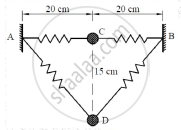Concept: Velocity and Acceleration in Terms of Rectangular Co-ordinate System
Chapter:  Kinematics of Particle
 5.d

Two balls, A (mass 3kg) and B (mass 4kg), are moving with velocities 25 m/s and 40 m/s respectively (Refer Figure 15). Before impact, the direction of velocity of two balls are 300 and 500 with the line joining their centers as shown in Figure 15. If coefficient of restitution for the impact is 0.78, find the magnitude and the direction of velocities of the balls after the impact.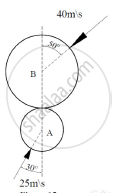Concept: Velocity and Acceleration in Terms of Rectangular Co-ordinate System
Chapter:  Kinematics of Particle
 6
 6.a

For the truss shown in Figure 16, find the forces in members DE, BD and CB.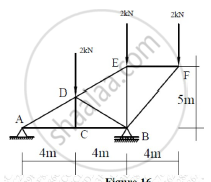Chapter:  Types of Support
 6.b

A particle moves in x-y plane with acceleration components ax = -3m/s2 and ay = -16t m/s2. If its initial velocity is V0 = 50m/s directed at 350 to the x–axis, compute the radius of curvature of the path at t = 2 sec.

Concept: Tangentialand Normal Component of Acceleration
Chapter:  Kinematics of Particle
 6.c

A force of magnitude of 20kN, acts at point A(3,4,5)m and has its line of action passing through B(5,-3,4)m. Calculate the moment of this force about a line passing through points S(2,-5,3) m and T(-3,4,6)m.

Concept: Moment of force about a point
Chapter:  System of Coplanar Forces
 6.d

Find an expression for maximum range of a particle which is projected with an initial velocity of ‘u’ inclined at an angle of ‘β’ with the horizontal.

Concept: Motion Curves (a-t, v-t, s-t curves)
Chapter:  Kinematics of Particle

#### Request Question Paper

If you dont find a question paper, kindly write to us

View All Requests

#### Submit Question Paper

Help us maintain new question papers on Shaalaa.com, so we can continue to help students

only jpg, png and pdf files

## University of Mumbai previous year question papers Semester 1 (FE First Year) Engineering Mechanics with solutions 2018 - 2019

University of Mumbai Semester 1 (FE First Year) Engineering Mechanics question paper solution is key to score more marks in final exams. Students who have used our past year paper solution have significantly improved in speed and boosted their confidence to solve any question in the examination. Our University of Mumbai Semester 1 (FE First Year) Engineering Mechanics question paper 2019 serve as a catalyst to prepare for your Engineering Mechanics board examination.
Previous year Question paper for University of Mumbai Semester 1 (FE First Year) Engineering Mechanics-2019 is solved by experts. Solved question papers gives you the chance to check yourself after your mock test.
By referring the question paper Solutions for Engineering Mechanics, you can scale your preparation level and work on your weak areas. It will also help the candidates in developing the time-management skills. Practice makes perfect, and there is no better way to practice than to attempt previous year question paper solutions of University of Mumbai Semester 1 (FE First Year).

How University of Mumbai Semester 1 (FE First Year) Question Paper solutions Help Students ?
• Question paper solutions for Engineering Mechanics will helps students to prepare for exam.
• Question paper with answer will boost students confidence in exam time and also give you an idea About the important questions and topics to be prepared for the board exam.
• For finding solution of question papers no need to refer so multiple sources like textbook or guides.